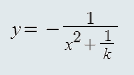All about flooble | fun stuff | Get a free chatterbox | Free JavaScript | Avatarsperplexus dot infoConcavity (Posted on 2014-08-26)For the function below, determine the range of x-values for which the function is concave up:

1) Assuming k is negative.
2) Assuming k is positive.No Solution Yet Submitted by Dustin Rating: 4.0000 (1 votes)Comments: ( Back to comment list | You must be logged in to post comments.)Solution Comment 2 of 2 |y = - (x2 + 1/k)-1

Differentiating:      y�      = 2x(x2 + 1/k)-2

and again:             y��     = 2(x2 + 1/k)-2 � 4x(x2 + 1/k)-32x

= 2(x2 + 1/k)-3(x2 + 1/k � 4x2)

= 2(x2 + 1/k)-3(1/k � 3x2)

(1)  For k < 0: 1/k � 3x2 < 0,  so the function will be concave upwards

(i.e. y�� > 0) iff  x2 + 1/k < 0, giving |x| < sqrt(-1/k).

i.e. between the asymptotes, x = +/-sqrt(-1/k), as Charlie found,

with a local minimum at (0, -k), above the origin.

(2)  For k>0:
(x2 + 1/k)-3 > 0, so the function will be concave upwards

where   1/k � 3x2 > 0, giving  |x| < 1/sqrt(3k).

i.e. between the points of inflection at (+/-1/sqrt(3k), -3k/4),

with a local minimum at (0, -k), below the origin.

 Posted by Harry on 2014-08-27 13:00:37Please log in:

 Search: Search body:
Forums (0)